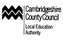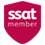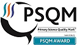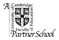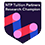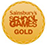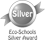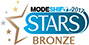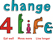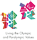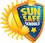# Learning Expectations in Year 6

By May in Year 6 children should be confident doing the following...

• I can apply knowledge of root words, prefixes and suffixes to read aloud and to understand the meaning of unfamiliar words.
• I use my combined knowledge of phonemes and word deriviations to pronounce words correctly, e.g. arachnophobia.
• I attempt the pronunciation of unfamiliar words drawing on my prior knowledge of similar looking words.
• I can read fluently, using punctuation to inform meaning.

• I am familiar with and can talk about a wide range of books and text types, including myths, legends and traditional stories and books from other cultures and traditions. I can discuss the features of each.
• I can read books that are structured in different ways.
• I can recognise texts that contain features from more than one text type.
• I can evaluate how effectively texts are structured and presented.
• I can read non-fiction texts to help with my learning.
• I read accurately and check that I understand.
• I can recommend books to others and give reasons for my recommendation. • I can identify themes in texts.
• I can identify and discuss the conventions in different text types.
• I can identify the key points in a text.
• I can recite a range of poems by heart, e.g. narrative verse, sonnet.
• I can prepare poems and plays to read aloud and to perform, showing understanding through intonation, tone, volume and action.

Spelling

• I can convert verbs into nouns by adding a suffix.
• I can distinguish between homophones and other words which are often confused.
• I can spell the commonly mis-spelt words from the Y5/6 word list.
• I understand that the spelling of some words need to be learnt specifically.
• I can use any dictionary or thesaurus.
• I use a range of spelling strategies.

Handwriting

• I can choose the style of handwriting to use when given a choice.
• I can choose the handwriting that is best suited for a specific task.

Sentence structure

• I can use the passive voice.
• I can vary sentence structure depending whether formal or informal.

Text structure

• I can use a variety of organisational and presentational devices correct to the text type.
• I write in paragraphs which can clearly signal a change in subject, time, place or event.

Punctuation

• I can use the semi-colon, colon and dash.
• I can use the colon to introduce a list and semi-colon within lists.
• I can use a hyphen to avoid ambiguity.

Number

• I can use negative numbers in context, and calculate intervals across zero.
• I can round any whole number to a required degree of accuracy and solve problems which require answers to be rounded to a specific degree of accuracy.
• I can solve problems involving the relative sizes of two quantities where the missing values can be found by using integer multiplication and division facts.
• I can use common factors to simplify fractions; use common multiples to express fractions in the same denomination.
• I can solve problems involving the calculation of percentages.
• I can multiply 1-digit numbers with up to two decimal places by whole numbers.
• I can perform mental calculations, including with mixed operations with large numbers.
• I can divide numbers up to 4-digits by a 2-digit whole number using formal written methods of long division and interpret remainder in various ways.
• I use my knowledge of order of operations to carry out calculations involving all four operations. • I can add and subtract fractions with different denominators and mixed numbers, using the concept of equivalent fractions.
• I can multiply simple pairs of proper fractions, writing the answer in its simplest form.
• I can divide proper fractions by whole numbers.
• I can associate a fraction with division and calculate decimal fraction equivalents.
• I can express missing number problems algebraically.
• I can find pairs of numbers that satisfy number sentences involving two unknowns.

Measurement, geometry and statistics

• I can recognise, describe and build simple 3D shapes, including making nets.
• I can compare and classify geometric shapes based on their properties and sizes and find unknown angles in any triangle, quadrilateral and regular polygons.
• I can illustrate and name parts of circles, including radius, diameter and circumference and know that the radius is half the diameter.
• I can read, write and convert between standard units, converting measurements of length, mass, volume and time from a smaller unit of measure to a larger unit, and visa versa, using decimal notation to up to 3 decimal places.
• I can calculate the area of a parallelogram and triangles and calculate, estimate and compare volume of cubes and cuboids using standard units.
• I can interpret and construct pie charts and line graphs and use these to solve problems.